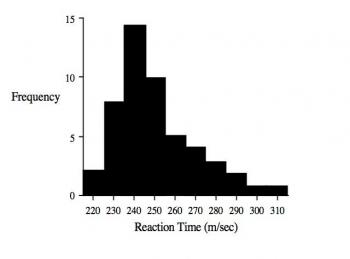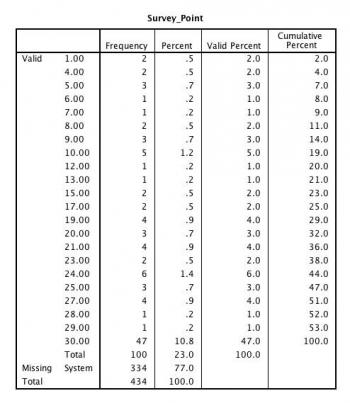# Chapter 6: Descriptive Statistics

1. A researcher conducts an experiment in which she assigns participants to one of two groups and exposes the two groups to different doses of a particular drug. She then gets the participants to learn a list of 20 words and two days later sees how many they can recall. In the experiment the dependent measure is simply the number of words recalled by each participant. What type of dependent measure is this? [TY6.1]

1. Nominal.
2. Ordinal.
3. Interval.
4. Ratio.
5. None of the above.

2. A researcher conducts a study to find out how many times people had visited a doctor in the previous year. Five people participated in the study and the numbers of visits they had made were 2, 5, 7, 4 and 2. Which of the following statements is true? [TY6.2]

1. The mean number of visits is 2.
2. The median number of visits is 2.
3. The median number of visits is 4.
4. The modal number of visits is 4.
5. The modal number of visits is 7.

3. Which of the following statements is most likely to be true if the distribution of a variable is severely skewed? [TY6.3]

1. The mean will be the best measure of central tendency.
2. The median will be as misleading as the mean.
3. The mode will no longer be the most common response.
4. The median will be the best measure of central tendency.
5. The mode will be the best measure of central tendency.

4. If X is a variable, which of the following is not measured in the same units as X? [TY6.4]

1. The mean of X.
2. The range of X.
3. The standard deviation of X.
4. The variance of X.
5. The difference between minimum and maximum values of X.

5. If scores on a variable are normally distributed, which of the following statements is false? [TY6.5]

1. All scores on the variable will have been observed with equal frequency.
2. The distribution of scores is symmetrical about the mean.
3. Similar distributions are commonly observed in data obtained from psychological research.
4. There will be relatively few extreme scores.
5. The mean, median and modal scores will be equal.

6. Which of the following is not a measure of central tendency? [TY6.6]

1. The mean of a distribution.
2. The mean squared deviation of some data.
3. The modal value of a set of values.
4. The median response on a scale.
5. An average score.

7. The shaded bars in the histogram below represent the times (rounded to the nearest 10 milliseconds) that 50 people take to react to a loud noise. Which of the following statements is not true?1. The distribution of reaction times is negatively skewed.
2. The modal reaction time is 240 ms.
3. The median reaction time is greater than 240 ms.
4. The mean reaction time will be greater than the modal reaction time.
5. The mean is an ambiguous measure of central tendency.

8. ‘Root mean squared deviate’ could be used as another name for which measure of dispersion? [TY6.8]

1. Range.
2. Skewness
3. Variance.
4. Standard deviation.
5. Squared deviation from the root.

9. A researcher measures a variable whose distribution she observes to be normally distributed. On this basis which of the following statements is most likely to be true?

1. The distribution's mean will be greater than its median and mode.
2. The distribution's median will be greater than its mean and mode.
3. The distribution's mean will be similar to its median and mode.
4. The distribution's mean will be less than its median and mode.
5. The distribution's mean will be greater than its median but less than its mode.

10. Which of the following is a measure of central tendency?

1. The variance in scores obtained on a dependent measure.
2. The mean deviation of some data.
3. Standard deviation.
4. The range of a set of values.
5. None of the above.

11. The SPSS output below is from a study in which the scores for the variable “Survey_Point” could vary between 0 and 30. Looking at the distribution of frequencies, which of the following statements is true?1. The distribution of scores is positively skewed.
2. The distribution of scores is negatively skewed.
3. There is a uniform distribution of scores.
4. The data have a bimodal distribution.
5. None of the above.

12. The SPSS output below is from a study in which the scores for the variable “Survey_Point” could vary between 0 and 30. Looking at the distribution of frequencies, which of the following statements is true?1. The mean will be higher than the mode.
2. The mode will be higher than the mean.
3. The mean will be the same as the mode.
4. The median will be lower than the mean.
5. The median will be higher than the mode.

13. “A variable that can be treated as if there were no breaks or steps between its different levels (e.g., reaction time in milliseconds).” What type of variable is this a glossary definition of?

1. A logical variable.
2. A discrete variable.
3. A scale variable.
4. A continuous variable.
5. A measurement variable.

14. “A measure reflecting distinct categories that have different names but the categories are not numerically related to one another.” What type of measure is this a glossary definition of?

1. An ordinal measure.
2. A nominal measure.
3. A scale measure.
4. A desperate measure.
5. A ratio measure.

15. “The spread of scores across levels of a variable.” What is this a glossary definition of?

1. Distribution.
2. Range.
3. Variance.
4. Covariance.
5. Standard deviation.